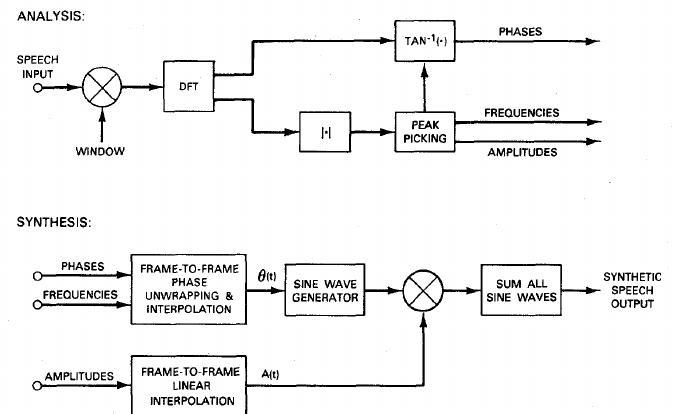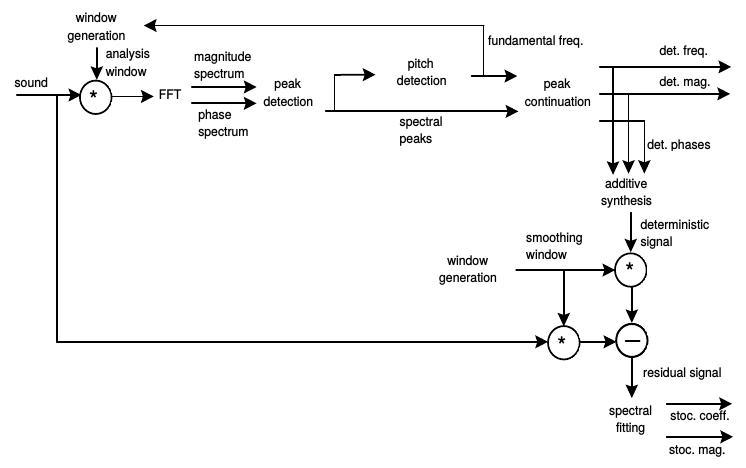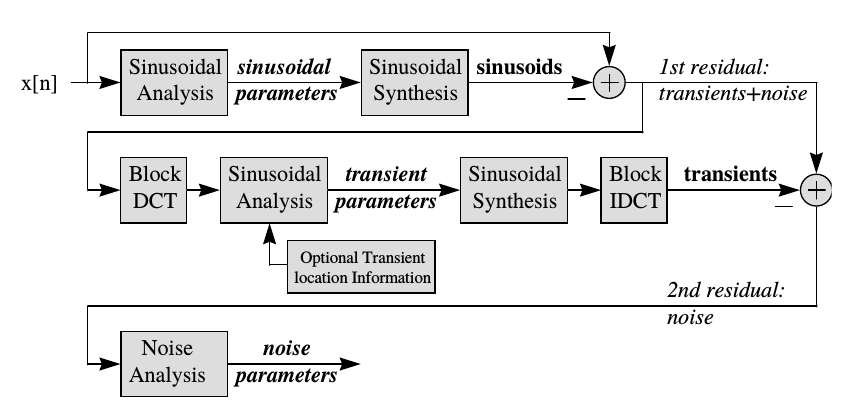# Additive & Spectral: Spectral Modeling

## McAulay/Quatieri

Sinusoidal modeling can be considered a higher level algorithm for the additive synthesis of harmonic sounds. It has first been used in speech processing by McAulay, R. and Quatieri (1986). For low framerates they proposed a time-domain method for partial synthesis with original phases of the partials.R. McAulay and T. Quatieri (1986)

## SMS

The above presented sinusoidal modeling approach captures only the harmonic portion of a sound. With the Sinusoids plus Noise model (SMS), Serra and Smith (1990) introduced the Deterministic + Stochastic model for spectral modeling, in order to model components in the signal which are not captured by partial tracking. A sound is therefor modeled as a combination of a dererministic component - the sinusoids - and a stochasctic component:

\begin{equation*} x = x_{DET} + x_{STO} \end{equation*}Deterministic + Stochastic model (Serra and Smith, 1990)

### Violin Example

The following example shows the sines + noise decomposition for a single violin sound. The original recording was made in an anechoic chamber:

After partial tracking, the deterministic component can be re-synthesized using an oscillator bank. It features the strings oscillation, in this case with original phases. For a bowed string instrument like the violin, the deterministic model alone can deliver plausible results:

The residual signal still carries some parts of the deterministic part, when calculated with simple subtraction. Most of the residual's energy is caused by the bow friction:

## Sines + Transients + Noise

Even the harmonic and noise model can not capture all components of musical sounds. The third - and in this line last - signal component to be included are the transients.Sines + Transients + Noise (Levine and Smith, 1998)